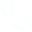# 【DSW Gallery】Tensorflow2 builds CNN model

Tensorflow2 And Keras
Tensorflow 2 is a deep learning framework developed by Google based on Tensorflow 1. In terms of architecture, API, and supported hardware types have been deeply optimized. The architecture of Tensorflow 2 mainly includes two layers
1. Training layer
2. Deployment layer
The main features of Tensorflow 2 - (1) use tf.data to load data - (2) use tf.keras to build models, or use premade estimators to verify models, use tensorflow hub for migration learning - (3) use eager mode to run And debugging - (4) Use distribution strategy for distributed training - (5) Export to SaveModel - (6) Use Tensorflow Server, TensorFlow Lite, TensorFlow.js to deploy models - (7) Powerful cross-platform capabilities, Tensorflow2 services directly Implemented through HTTP/REST or GRPC/protocol buffer, TensorFlow Lite can be directly deployed on Android, IOS and embedded systems, TensorFlow.js deploys models in javascript - (8) Tf.keras function API and subclass API, allowing Create a responsible topology - (9) Customize training logic, use tf.GradientTape and tf.custom_gradient for finer-grained control - (10) Low-level API can be used in combination with high-level, fully customizable - (11) Advanced extension : Ragged Tensors, Tensor2Tensor
Keras Keras is a high-level API of Tensorflow, which can effectively improve the efficiency of model development
1. Import Tensorflow
import tensorflow as tf
import seaborn as sns
import numpy as np
import pandas as pd
import matplotlib.pyplot as plt
import matplotlib.image as mpimg
mnist = tf.keras.datasets.mnist
(x_train, y_train), (x_test, y_test) = mnist. load_data()
11493376/11490434 [=================================] - 0s 0us/step
2.1 Visually look at the current data set, whether the samples of each category are balanced
sns. countplot(y_train)
/home/pai/lib/python3.6/site-packages/seaborn/_decorators.py:43: FutureWarning: Pass the following variable as a keyword arg: x. From version 0.12, the only valid positional argument will be `data` , and passing other arguments without an explicit keyword will result in an error or misinterpretation.
FutureWarning

2.2 Check if there are NaN samples in the training data
np.isnan(x_train).any()
False
Check if there are Nan samples in the test data set
np.isnan(x_test).any()
False
3. Data preprocessing, do two things here:
a. reshape our input data set to meet the requirements of the input data shape of the model in this paper
b. Normalization
input_shape = (28, 28, 1)
x_train=x_train.reshape(x_train.shape, x_train.shape, x_train.shape, 1)
x_train=x_train / 255.0
x_test = x_test.reshape(x_test.shape, x_test.shape, x_test.shape, 1)
x_test=x_test/255.0
Encode the label, here use one-hot-encoding
y_train = tf.one_hot(y_train.astype(np.int32), depth=10)
y_test = tf.one_hot(y_test.astype(np.int32), depth=10)
4. Building a CNN model
a. Use the tf.keras.models.Sequential interface to build
b. Add convolution layer tf.keras.layers.Conv2D in turn
c. MaxPooling layer tf.keras.layers.MaxPool2D
d. Dropout tf.keras.layers.Dropout
e. Fully connected layer tf.keras.layers.Dense
batch_size = 64
num_classes = 10
epochs = 50
model = tf.keras.models.Sequential([
tf.keras.layers.MaxPool2D(),
tf.keras.layers.Dropout(0.25),
tf.keras.layers.MaxPool2D(strides=(2,2)),
tf.keras.layers.Dropout(0.25),
tf.keras.layers.Flatten(),
tf.keras.layers.Dense(128, activation='relu'),
tf.keras.layers.Dropout(0.5),
tf.keras.layers.Dense(num_classes, activation='softmax')
])
model.compile(optimizer=tf.keras.optimizers.RMSprop(epsilon=1e-08), loss='categorical_crossentropy', metrics=['acc'])
5. Define related callback functions
• This callback function is to check whether the accuracy is greater than 99.5% at the end of each epoch, and if so, stop training
class myCallback(tf.keras.callbacks.Callback):
def on_epoch_end(self, epoch, logs={}):
if(logs.get('acc')>0.995):
print(" Reached 99.5% accuracy so canceling training!")
self.model.stop_training=True
callbacks = myCallback()

## Related Articles

• ### A detailed explanation of Hadoop core architecture HDFS

Knowledge Base Team

• ### What Does IOT Mean

Knowledge Base Team

• ### 6 Optional Technologies for Data Storage

Knowledge Base Team

• ### What Is Blockchain Technology

Knowledge Base Team

## Explore More Special Offers

1. ### Short Message Service(SMS) & Mail Service

50,000 email package starts as low as USD 1.99, 120 short messages start at only USD 1.00Contact Us